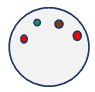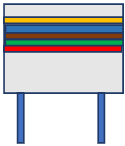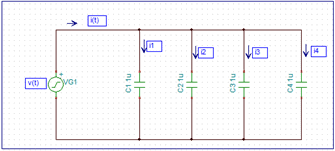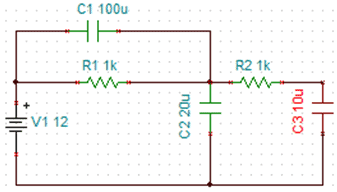# Capacitors MCQs with Explanatory Answers

## Capacitors MCQs with Explanatory Answers

1.  The Mark on Ceramic or Non-Polarized Capacitors is “104”. What is the value of Capacitor?

1. 104 µF
2. 10000 µF
3. 10000 nF
4. 100000 pF

Explanation:
Here is the Capacitor marking is “104”
It’s mean that = 10 + 4 Zeros = 1,000,00 pF
= 100 nF = 0.1 µF

2.  A capacitor passes AC and blocks DC

1. True
2. False

Capacitive Reactance is given as:

Xc = 1/2 πfC

Where, f is the frequency of applied voltage to the capacitor plates and C is the capacitance.

For DC, the value of f is zero and hence the capacitive reactance is infinite. Since capacitive reactance denotes the opposition to flow of current just like resistance, this implies the capacitor blocks passage of DC current.

Since frequency of AC signals is anything but zero or less than that, capacitive reactance has a finite value for AC supply and allows to pass AC.

3.  Leads of all capacitors are polarized.

1. True
2. False

Capacitors are of two types based on their polarity – Electrolyte and Non-Polarized Capacitors. While Electrolyte capacitors have polarized leads, the non-polarized capacitors like ceramic, mica etc. have equivalent leads.

4.  Value of the below disk ceramic capacitor is:1.   400pF,  +/- 5%  tolerance
2.   250pF,  +/- 2% tolerance
3.   300pF,  +/- 4% tolerance
4.   100nF,  +/- 2%  tolerance

As per the colour code for disk ceramic capacitor, the first two colours represent the value of capacitance, the third colour represents the multiplier and the last colour represents the tolerance.

Thus, referring the colour chart, we come to the following conclusion:

1. First colour: Red. Hence first digit is 2
2. Second colour: Green. Hence second digit is 5
3. Thus, value of capacitance is 25
4. Third colour: Brown. Hence multiplier is 10pF
5. Fourth colour: Red. Hence tolerance is +/-2%

Therefore, value of the capacitor is 25 x 10pF, +/-2%,   i.e.  250pF, +/-2%  tolerance.

5.  Reading of the below capacitor is1.  46  x 10pF,   +/-5% Tolerance, 250  Volts
2.  50  x 100pF, +/-2% Tolerance, 100  Volts
3.  35  x 10uF,   +/-1% Tolerance,  60   Volts
4.  50  x 100nF, +/-4% Tolerance,  250 Volts

As per the colour code for tubular capacitor, the first two colours represent the value of capacitance, the third colour represents the multiplier, fourth colour represents the tolerance and last colour represents the maximum permissible voltage across the capacitor.

Thus, referring the colour chart, we come to the following conclusion:

1.   First colour:       Yellow. Hence first digit is 4
2.   Second colour:   Blue. Hence second digit is 6
3.   Thus, value of capacitance is 46.
4.   Third colour:      Brown. Hence multiplier is 10pF
5.   Fourth colour:   Green. Hence tolerance is +/-5%
6.   Fifth colour:       Red. Hence voltage is 250 Volts

Thus, value of the capacitor is: 46 x 10pF, +/-5% tolerance, 250 Volts.

6.  For capacitors connected in parallel, the total capacitance would be:

1. Product of the individual capacitances
2. Sum of the individual capacitances
3. Inverse of the sum of inverse values of capacitors
4. None of these

Consider the below parallel connection of capacitorsApplying Kirchhoff’s current law, we getNow we know current through capacitor N is,Also, voltage across each capacitor is same.

Substituting in equation 1, we get:Thus, for parallel connection of capacitors, the total capacitance is the summation of individual capacitances.

7.  For capacitors connected in series, the total capacitance is:

1. Same as that of value of total resistances in parallel connection
2. Sum of the individual values
3. Same as that of value of total resistances in series
4. None of the these

Consider the circuit given belowApplying Kirchhoff’s voltage law, we get:Now, voltage across capacitor N,Since current through the loop is same. Hence equation 1 becomes:Thus, total capacitance for capacitors in series is same as total resistances in parallel.

8.  Energy stored in the capacitor is:

1.    ¼ CV
2.    ½ CV2
3.    CV2
4.    1/2CV

When voltage, v(t) is applied to a capacitor, current I flows through it. The energy stored in the capacitor is the integral of the instantaneous power.

Thus, energy stored in capacitor, E = 1/2CV2

9.  Value of ESR for actual capacitors are in the range of:

1. Milli Ohms to Ohms
2. Micro Ohms to Milli Ohms
3. Ohms to Mega Ohms
4. Nano Ohms to Ohms

Even though theoretically the dielectric material between plates of a capacitor has infinite resistivity, it has some finite resistivity which leads to flow of current known as leakage current. The Equivalent Series Resistance (ESR) is the total resistance offered by the dielectric material and other metal elements in the capacitor and denotes the total losses incurred. It typically ranges from mili-ohms to ohms.

10.  For the below circuit, energy stored in individual capacitors at DC conditions is:1.   0 Joules, 12 x 10^- 4 Joules, 72 x 10-4 Joules
2.   0 Joules, 144 x 10-5 Joules, 72 x 10-5 Joules
3.   72 x 10-4 Joules, 144 micro Joules, 0 Joules
4.   None of these

Given: Voltage across capacitor, C1 = 0

Hence energy stored in capacitor, C1 = 0 Joules

Voltage across capacitor, C2 = 12V

Hence, energy stored in capacitor, C2 = 1/ 2CV2 = 144 x 10-5 Joules

Voltage across capacitor, C3 = 12V

Hence, energy stored in capacitor, C3 = 1/ 2CV2 = 72 x 10-5 Joules

11.  For the below circuit, current through the capacitor is———-?1.  0.2cos    100πt
2.  0.15cos  100πt
3.  0.1cos    100πt
4.  0.25cos  100πt

Given:

Voltage, v(t) = 30sin ( 100 πt )

Capacitance, C1 = 50uF

Thus current through capacitor, i(t) = Cdv (t)/ dt = 50 x 10-6 d( 30sin 100πt ) /dt = 50 x 10-6 x 30 x 100 x πcos 100 πt = 15 x 10-2cos 100πt

12.  A capacitor works in an———–

1.   Electric Field
2.   Magnetic Field
3.   None of these
4.   Both

A capacitor consists of two electric conductors separated by a distance d with a dielectric material. When voltage is applied to the conductors, the potential difference between the conductors charges the conductors, which creates a uniform electric field between the conductors. This electric field is defined as the force per unit charge. Thus, a capacitor works in electric field.

13.  Capacitance of a capacitor is the measure of its ability to ——-

1. Resist flow of current
2. Store charge
3. Allow flow of current
4. None of these

Capacitance is the measure of a capacitor to store charge for a given electric potential. If it charged to ‘V’ volts, it stores charge +Q at one plate and charge -Q at another plate. Capacitance of 1 Farad denotes storage of 1 Coulomb charge for 1 volt potential difference.

14.  When DC voltage is applied to a capacitor, it———–

1. Acts as a short circuit at the instance of applied voltage and then as an open circuit once fully charged
2. Acts as an open circuit from the beginning
3. Acts as a short circuit
4. None of these

Consider the circuit given below:When the DC voltage is applied to the capacitor, initially high current passes through the capacitor plates as it starts getting charged. After time ‘t’, the value of current drops to zero as the capacitor plates get fully charged. This time ‘t’ is given as the reciprocal of RC, where R is the value of resistor R1.

15.  Capacitors are used in electric power supply system to——–

1. Improve power factor
2. Reduce line current
3. Provide voltage stability
4. All of these

Most of the electric power supply systems have inductive loads, which causes lagging effect on the supply current. This causes an increase in apparent or reactive power and increases the supply current.

### Electrical Technology

1. Md.Arif says:

[* WordPress Simple Firewall plugin marked this comment as “trash” because: Failed GASP Bot Filter Test (comment token failure). *]
[* WordPress Simple Firewall plugin marked this comment as “trash” because: Failed GASP Bot Filter Test (comment token failure). *]
Assalamualikum brother…In ur site we r nt getting ur answers for the questions..please rectify ur site sooon..it is very helpful to us..hope u understand…jazak allah khirun..

2. Mahesh Ganganmale says:

Godd I liked it..
Thank you 😍🤩🎉🔥

Close

Close